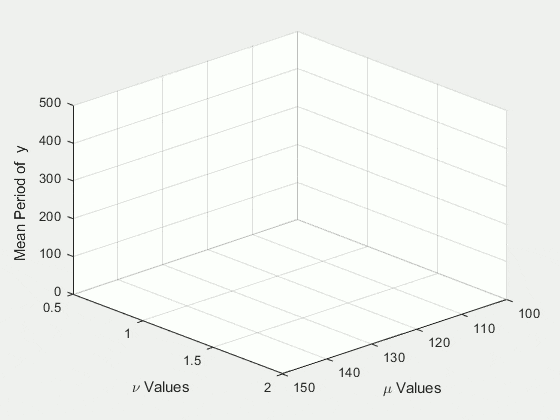# Plot During Parameter Sweep with parfor

This example shows how to perform a parameter sweep in parallel and plot progress during parallel computations. You can use a DataQueue to monitor results during computations on a parallel pool. You can also use a DataQueue with parallel language features such as parfor, parfeval and spmd.

The example shows how to perform a parameter sweep on a classical system, the Van der Pol oscillator. This system can be expressed as a set of ODEs dependent on the two Van der Pol oscillator parameters, $\mu$ and $\nu$:

$\underset{}{\overset{˙}{x}}=\nu y$

$\underset{}{\overset{˙}{y}}=\mu \left(1-{x}^{2}\right)y-x$

You can perform a parallel parameter sweep over the parameters $\mu$ and $\nu$ using a parfor loop to find out the mean period of $y$ when varying them. The following animation shows an execution of this example in a local cluster.### Set Up Parameter Sweep Values

Define the range of values for the parameters to be explored. Create a meshgrid to account for the different combinations of the parameters.

gridSize = 6; mu = linspace(100, 150, gridSize); nu = linspace(0.5, 2, gridSize); [M,N] = meshgrid(mu,nu);

### Prepare a Surface Plot to Visualize the Results

Declare a variable to store the results of the sweep. Use nan for preallocation to avoid plotting an initial surface. Create a surface plot to visualize the results of the sweep for each combination of the parameters. Prepare settings such as title, labels, and limits.

Z = nan(size(N)); c = surf(M, N, Z); xlabel('\mu Values','Interpreter','Tex') ylabel('\nu Values','Interpreter','Tex') zlabel('Mean Period of y') view(137, 30) axis([100 150 0.5 2 0 500]);### Set Up a DataQueue to Fetch Results During the Parameter Sweep

Create a DataQueue to send intermediate results from the workers to the client. Use the afterEach function to define a callback in the client that updates the surface each time a worker sends the current result.

D = parallel.pool.DataQueue; D.afterEach(@(x) updateSurface(c, x));

### Perform the Parameter Sweep and Plot Results

Use parfor to perform a parallel parameter sweep. Instruct the workers to solve the system for each combination of the parameters in the meshgrid, and compute the mean period. Immediately send the result of each iteration back to the client when the worker finishes computations.

parfor ii = 1:numel(N) [t, y] = solveVdp(M(ii), N(ii)); l = islocalmax(y(:, 2)); send(D, [ii mean(diff(t(l)))]); end
Starting parallel pool (parpool) using the 'local' profile ... Connected to the parallel pool (number of workers: 6). 

### Scale Up to a Cluster

If you have access to a cluster, you can scale up your computation. To do this, delete the previous parpool, and open a new one using the profile for your larger cluster. The code below shows a cluster profile named 'MyClusterInTheCloud'. To run this code yourself, you must replace 'MyClusterInTheCloud' with the name of your cluster profile. Adjust the number of workers. The example shows 4 workers. Increase the size of the overall computation by increasing the size of the grid.

gridSize = 25; delete(gcp('nocreate')); parpool('MyClusterInTheCloud',4);
Starting parallel pool (parpool) using the 'MyClusterInTheCloud' profile ... Connected to the parallel pool (number of workers: 4). 

If you run the parameter sweep code again after setting the cluster profile, then the workers in the cluster compute and send the results to the MATLAB client when they become available. The following animation shows an execution of this example in a cluster.### Helper Functions

Create a helper function to define the system of equations, and apply the solver on it.

function [t, y] = solveVdp(mu, nu) f = @(~,y) [nu*y(2); mu*(1-y(1)^2)*y(2)-y(1)]; [t,y] = ode23s(f,[0 20*mu],[2; 0]); end

Declare a function for the DataQueue to update the graph with the results that come from the workers.

function updateSurface(s, d) s.ZData(d(1)) = d(2); drawnow('limitrate'); end